##### The Japanese Maple specialist
Direct order Contact Help / Services Newsletter# Shelf to displaySearch

Japanese MaplesAcer seeds and more Young maples and bonsaiHostasWind bells and chimesSaxifragesVarieties introduced into Europe

Packing and shipping charges

# Ryu sei

› Japanese maples › Acer palmatum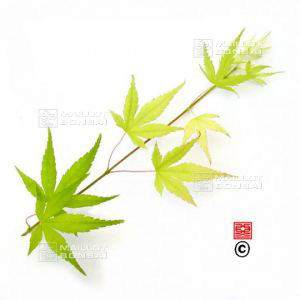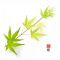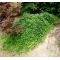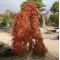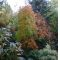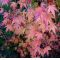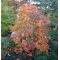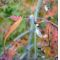ref. : 868

Choose the right size
Pot size

This item is temporarily unavailable

###### Description

Foliage of yellow green spring becoming then green dark in summer orange and red in fall. Port naturally very falling again. Often transplanted on stem to take advantage of his/her/its port in cascade. Named as millennium green and ryu sen.

#green 3.5 #palmatum 2.8 #acer 2.4 #port 2.4 #transplanted 2.2 #millennium 2 #advantage 1.9 #naturally 1.9 #becoming 1.8 #cascade 1.7

###### Technical description
 Species Acer palmatum same species items Variety Ryu sei Leaf colour Green leaves same colour items Leaf shape Palmated same shaped items Adult height same height items Port Semi-spread same port items Expositions Sun same exposition items

Formule
(( ROUND((CHAR_LENGTH(b.article_nom)-CHAR_LENGTH(REPLACE(b.article_nom, 'green', '')))/LENGTH('green')) + ROUND((CHAR_LENGTH(b.article_description)-CHAR_LENGTH(REPLACE(b.article_description, 'green', '')))/LENGTH('green')) ) * 3.5) + (( ROUND((CHAR_LENGTH(b.article_nom)-CHAR_LENGTH(REPLACE(b.article_nom, 'port', '')))/LENGTH('port')) + ROUND((CHAR_LENGTH(b.article_description)-CHAR_LENGTH(REPLACE(b.article_description, 'port', '')))/LENGTH('port')) ) * 2.4) + (( ROUND((CHAR_LENGTH(b.article_nom)-CHAR_LENGTH(REPLACE(b.article_nom, 'transplanted', '')))/LENGTH('transplanted')) + ROUND((CHAR_LENGTH(b.article_description)-CHAR_LENGTH(REPLACE(b.article_description, 'transplanted', '')))/LENGTH('transplanted')) ) * 2.2) + (( ROUND((CHAR_LENGTH(b.article_nom)-CHAR_LENGTH(REPLACE(b.article_nom, 'millennium', '')))/LENGTH('millennium')) + ROUND((CHAR_LENGTH(b.article_description)-CHAR_LENGTH(REPLACE(b.article_description, 'millennium', '')))/LENGTH('millennium')) ) * 2) + (( ROUND((CHAR_LENGTH(b.article_nom)-CHAR_LENGTH(REPLACE(b.article_nom, 'advantage', '')))/LENGTH('advantage')) + ROUND((CHAR_LENGTH(b.article_description)-CHAR_LENGTH(REPLACE(b.article_description, 'advantage', '')))/LENGTH('advantage')) ) * 1.9) + (( ROUND((CHAR_LENGTH(b.article_nom)-CHAR_LENGTH(REPLACE(b.article_nom, 'naturally', '')))/LENGTH('naturally')) + ROUND((CHAR_LENGTH(b.article_description)-CHAR_LENGTH(REPLACE(b.article_description, 'naturally', '')))/LENGTH('naturally')) ) * 1.9) + (( ROUND((CHAR_LENGTH(b.article_nom)-CHAR_LENGTH(REPLACE(b.article_nom, 'becoming', '')))/LENGTH('becoming')) + ROUND((CHAR_LENGTH(b.article_description)-CHAR_LENGTH(REPLACE(b.article_description, 'becoming', '')))/LENGTH('becoming')) ) * 1.8) + (( ROUND((CHAR_LENGTH(b.article_nom)-CHAR_LENGTH(REPLACE(b.article_nom, 'falling', '')))/LENGTH('falling')) + ROUND((CHAR_LENGTH(b.article_description)-CHAR_LENGTH(REPLACE(b.article_description, 'falling', '')))/LENGTH('falling')) ) * 1.7) + (( ROUND((CHAR_LENGTH(b.article_nom)-CHAR_LENGTH(REPLACE(b.article_nom, 'foliage', '')))/LENGTH('foliage')) + ROUND((CHAR_LENGTH(b.article_description)-CHAR_LENGTH(REPLACE(b.article_description, 'foliage', '')))/LENGTH('foliage')) ) * 1.7) + (( ROUND((CHAR_LENGTH(b.article_nom)-CHAR_LENGTH(REPLACE(b.article_nom, 'cascade', '')))/LENGTH('cascade')) + ROUND((CHAR_LENGTH(b.article_description)-CHAR_LENGTH(REPLACE(b.article_description, 'cascade', '')))/LENGTH('cascade')) ) * 1.7)

## Secure payment## Delivery

Our logistic partners :